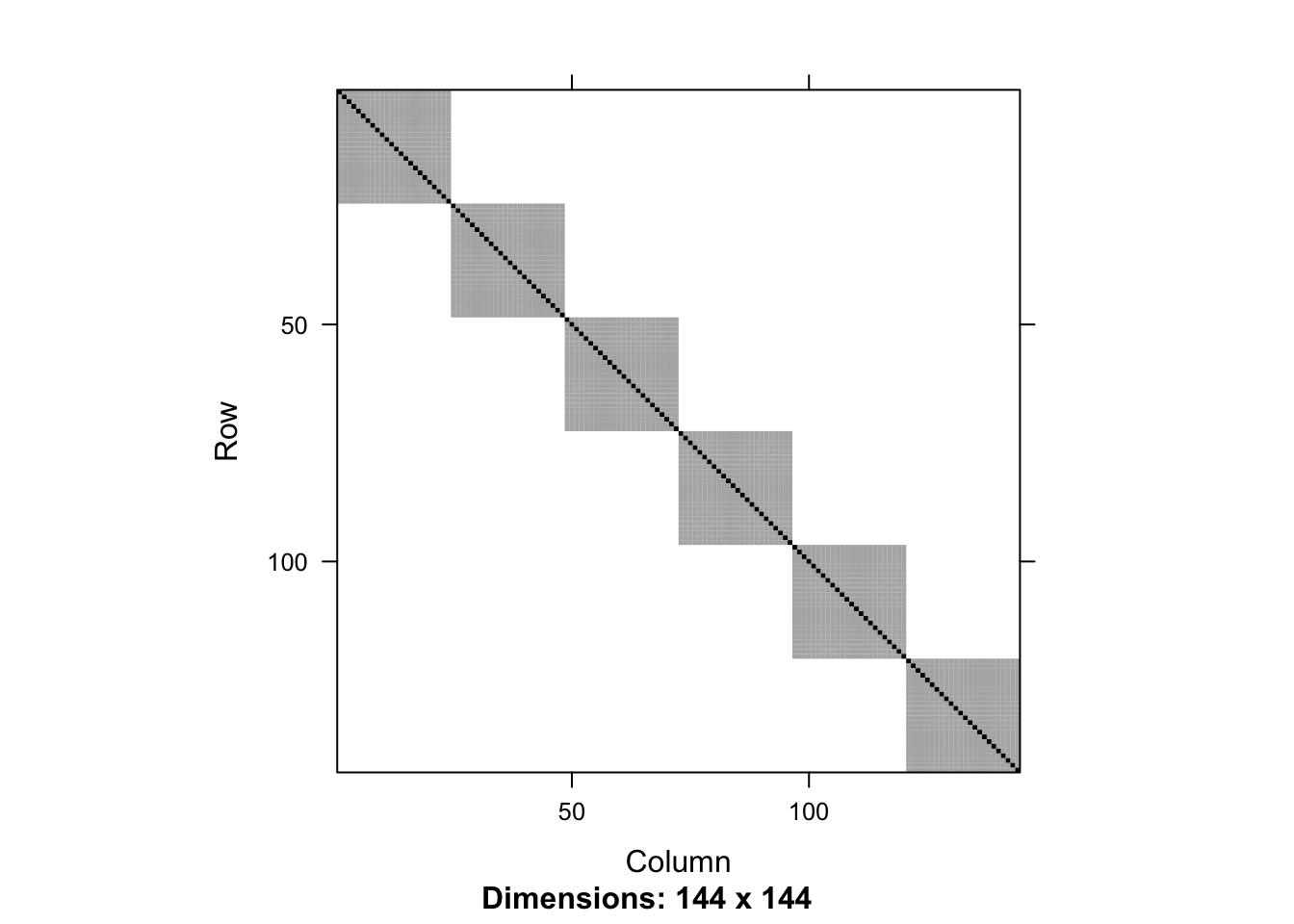# Random Effects

One way to think about random intercepts in a mixed models is the impact they will have on the residual covariance matrix. Of course, in a model with only fixed effects (e.g. `lm`), the residual covariance matrix is diagonal as each observation is assumed independent. In mixed models, there is a dependence structure across observations, so the residual covariance matrix will no longer be diagonal.

## Nested versus Crossed

Whether random effects are nested or crossed1 is a property of the data, not the model. However, when fitting the model, effects can be included as either nested or crossed.

Nested random effects are when each member of one group is contained entirely within a single unit of another group. The canonical example is students in classrooms; you may have repeated measures per student, but each student belongs to a single classroom (assuming no reassignments).

Crossed random effects are when this nesting is not true. An example would be different seeds and different fields used for planting crops. Seeds of the same type can be planted in different fields, and each field can have multiple seeds in it.

# The visualization function

This function extracts components of the mixed model and constructs the covariance matrix. From https://stackoverflow.com/a/45655597/905101

``````rescov <- function(model, data) {
var.d <- crossprod(getME(model,"Lambdat"))
Zt <- getME(model,"Zt")
vr <- sigma(model)^2
var.b <- vr*(t(Zt) %*% var.d %*% Zt)
sI <- vr * Diagonal(nrow(data))
var.y <- var.b + sI
invisible(var.y)
}``````

# Single random effect

The data is `Penicillin` from the “lmer” package.

``library(lme4)``
``## Loading required package: Matrix``
``````##
## Attaching package: 'Matrix'``````
``````## The following object is masked from 'package:tidyr':
##
##     expand``````
``````data(Penicillin)
``````##    diameter plate sample
## 1        27     a      A
## 2        23     a      B
## 3        26     a      C
## 4        23     a      D
## 5        23     a      E
## 6        21     a      F
## 7        27     b      A
## 8        23     b      B
## 9        26     b      C
## 10       23     b      D``````

This data is measuring Penicillin over a number of different trials. There are 24 plates and 6 samples, each plate having 1 replicate from each sample. (This is a fully crossed design, but it need not be.)

For the time being, let’s ignore the plate level effects, and fit a model with a random intercept only for sample.

In this data there are no covariates to enter as fixed effects, but their existence would not impede things.

``````mod1 <- lmer(diameter ~ 1 + (1 | sample), data = Penicillin %>% arrange(sample))
rc1 <- rescov(mod1, Penicillin)
image(rc1)``````(The data is re-ordered by `sample` to improve visualization. You generally want the data sorted first by the higher level, then within that level, the next highest level, etc.)

You see that this is block diagonal, with 6 blocks, each corresponding to one of the samples. This implies that the repeated measurements within each sample is correlated, but between samples are not correlated (as we expect).

# Cross random effects

Now let’s refit the above model, including the crossed random effets. In `lmer`, we simply add a second random intercept.

``````mod2 <- lmer(diameter ~ 1 + (1 | sample) + (1 | plate), data = Penicillin)
rc2 <- rescov(mod2, Penicillin)
image(rc2)``````